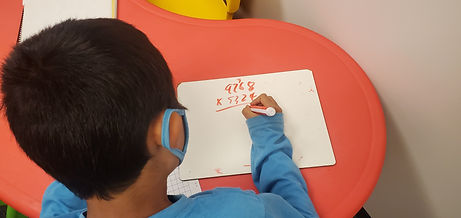## Mr. Jake

### Target 1​

###### Lesson Type:

Review

Number Operation

:

Computation

Multiply within 1,000.

###### 1:

Understand there are many ways to multiply multi-digit numbers.

###### 2:

Explain how different methods produce the same product (how each method is doing the same thing).

###### 3:

Understand the role of place-value in multiplication strategies.

###### 4:

Use the standard algorithm for solving multi-digit multiplication equations.

5th

###### Vocabulary:

Multiplication, Factor, Product

Activities:

Practiced solving multiplication problems with multiple digit numbers

Focused on finding their own errors and determining what went wrong in the problem solving process### Home Exploration

###### Guiding Questions:## Absent Students:

### Target 2

:

###### 1:

Describe the relationship between fractions, decimals, and percentages.

###### 2:

Convert fractions to decimals and decimals to fractions.

###### 3:

Understand that the digit to the left of the decimal is the whole number, the digits to the right are the numerator, and the denominator is the place-value of the furthest right number.

###### 4:

Identify the fractional equivalent of a percent.

6th

###### Vocabulary:

Fraction, Percent, Decimal, Convert

Activities:

Converted between fractions, decimals, and percents

Discussed how the different number representations are equal to one another### Home Exploration

###### Guiding Questions:### Target 3

:

###### Vocabulary:

Activities:### Home Exploration